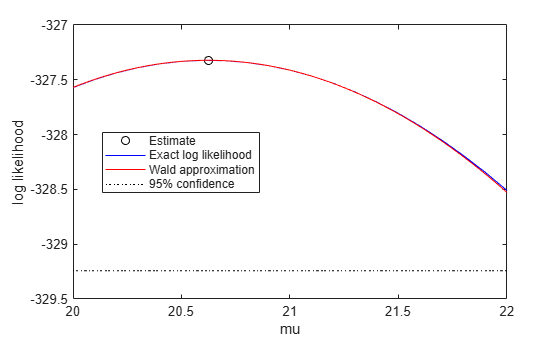# proflik

Profile likelihood function for probability distribution

## Syntax

``````[ll,param] = proflik(pd,pnum)``````
``````[ll,param] = proflik(pd,pnum,'Display',display)``````
``````[ll,param] = proflik(pd,pnum,setparam)``````
``````[ll,param] = proflik(pd,pnum,setparam,'Display',display)``````
``````[ll,param,other] = proflik(___)``````

## Description

example

``````[ll,param] = proflik(pd,pnum)``` returns a vector `ll` of loglikelihood values and a vector `param` of corresponding parameter values for the parameter in the position indicated by `pnum`.```
``````[ll,param] = proflik(pd,pnum,'Display',display)``` returns the loglikelihood values and corresponding parameter values, and plots the profile likelihood overlaid on an approximation of the loglikelihood.```
``````[ll,param] = proflik(pd,pnum,setparam)``` returns the loglikelihood values and corresponding parameter values as specified by `setparam`.```

example

``````[ll,param] = proflik(pd,pnum,setparam,'Display',display)``` returns the loglikelihood values and corresponding parameter values as specified by `setparam`, and plots the profile likelihood overlaid on an approximation of the loglikelihood.```

example

``````[ll,param,other] = proflik(___)``` also returns a matrix `other` containing the values of the other parameters that maximize the likelihood, using any of the input arguments from the previous syntaxes.```

## Examples

collapse all

Load the sample data. Create a probability distribution object by fitting a Weibull distribution to the miles per gallon (`MPG`) data.

```load carsmall pd = fitdist(MPG,'Weibull')```
```pd = WeibullDistribution Weibull distribution A = 26.5079 [24.8333, 28.2954] B = 3.27193 [2.79441, 3.83104] ```

View the parameter names for the distribution.

`pd.ParameterNames`
```ans = 1x2 cell {'A'} {'B'} ```

For the Weibull distribution, `A` is in position 1, and `B` is in position 2.

Compute the profile likelihood for `B`, which is in position `pnum = 2`.

`[ll,param] = proflik(pd,2);`

Display the loglikelihood values for the estimated values of `B`.

`[ll',param']`
```ans = 21×2 -329.9688 2.7132 -329.4312 2.7748 -328.9645 2.8365 -328.5661 2.8981 -328.2340 2.9597 -327.9658 3.0213 -327.7596 3.0830 -327.6135 3.1446 -327.5256 3.2062 -327.4943 3.2678 ⋮ ```

These results show that the profile log likelihood is maximized between the estimated `B` values of 3.2678 and 3.3295, which correspond to loglikelihood values -327.4943 and -327.5178. From the earlier fit, the MLE of `B` is 3.27193, which is in this interval as expected.

Load the sample data. Create a probability distribution object by fitting a generalized extreme value distribution to the miles per gallon (`MPG`) data.

```load carsmall pd = fitdist(MPG,'GeneralizedExtremeValue')```
```pd = GeneralizedExtremeValueDistribution Generalized Extreme Value distribution k = -0.207765 [-0.381674, -0.0338563] sigma = 7.49674 [6.31755, 8.89604] mu = 20.6233 [18.8859, 22.3606] ```

View the parameter names for the distribution.

`pd.ParameterNames`
```ans = 1x3 cell {'k'} {'sigma'} {'mu'} ```

For the generalized extreme value distribution, `k` is in position 1, `sigma` is in position 2, and `mu` is in position 3.

Compute the profile likelihood for `mu`, which is in position `pnum = 3`. Restrict the computation to parameter values from 20 to 22, and display the plot.

`[ll,param,other] = proflik(pd,3,20:.1:22,'display','on');`The plot shows the estimated value for the parameter `mu` that maximizes the loglikelihood.

Display the loglikelihood values for the estimated values of `mu`, and the values of the other distribution parameters that maximize the corresponding loglikelihood.

`[ll',param',other]`
```ans = 21×4 -327.5706 20.0000 -0.1803 7.4087 -327.4971 20.1000 -0.1846 7.4218 -327.4364 20.2000 -0.1890 7.4354 -327.3887 20.3000 -0.1934 7.4493 -327.3538 20.4000 -0.1978 7.4636 -327.3317 20.5000 -0.2023 7.4783 -327.3223 20.6000 -0.2067 7.4932 -327.3257 20.7000 -0.2112 7.5084 -327.3418 20.8000 -0.2156 7.5240 -327.3706 20.9000 -0.2201 7.5399 ⋮ ```

The first column contains the log likelihood value that corresponds to the estimate of `mu` in the second column. The log likelihood is maximized between the parameter values 20.6000 and 20.7000, corresponding to log likelihood values -327.3223 and -327.3257. The third column contains the value of `k` that maximizes the corresponding log likelihood for `mu`. The fourth column contains the value of `sigma` that maximizes the corresponding log likelihood for `mu`.

## Input Arguments

collapse all

Probability distribution, specified as a probability distribution object created using one of the following.

Function or AppDescription
`fitdist`Fit a probability distribution object to sample data.
Distribution FitterFit a probability distribution to sample data using the interactive Distribution Fitter app and export the fitted object to the workspace.

Parameter number for which to compute the profile likelihood, specified as a positive integer value corresponding to the position of the desired parameter in the parameter name vector. For example, a Weibull distribution has a parameter name vector `{'A','B'}`, so specify `pnum` as `2` to compute the profile likelihood for `B`.

Data Types: `single` | `double`

Parameter value restriction, specified as a scalar value or a vector of such values. If you do not specify `setparam`, `proflik` chooses the values for output vector `param` based on the default confidence interval method for the probability distribution `pd`. If the parameter can take only restricted values, and if the confidence interval violates that restriction, you can use `setparam` to specify valid values.

Example: `[3,3.5,4]`

Display toggle, specified as either `'on'` or `'off'`. Specify `'on'` to display the profile of the exact loglikelihood overlaid on the Wald approximation of the loglikelihood. Specify `'off'` to omit the display. The Wald approximation is based on a Taylor series expansion around the estimated parameter value, as a function of the parameter in position `pnum` or its logarithm. The intersection of the curves with the horizontal dotted line marks the endpoints of 95% confidence intervals.

## Output Arguments

collapse all

Loglikelihood values, returned as a vector. The loglikelihood is the value of the likelihood with the parameter in position `pnum` set to the values in `param`, maximized over the remaining parameters.

Parameter values corresponding to the loglikelihood values in `ll`, returned as a vector. If you specify parameter values using `setparam`, then `param` is equal to `setparam`.

Other parameter values that maximize the likelihood, returned as a matrix. Each row of `other` contains the values for all parameters except the parameter in position `pnum`.